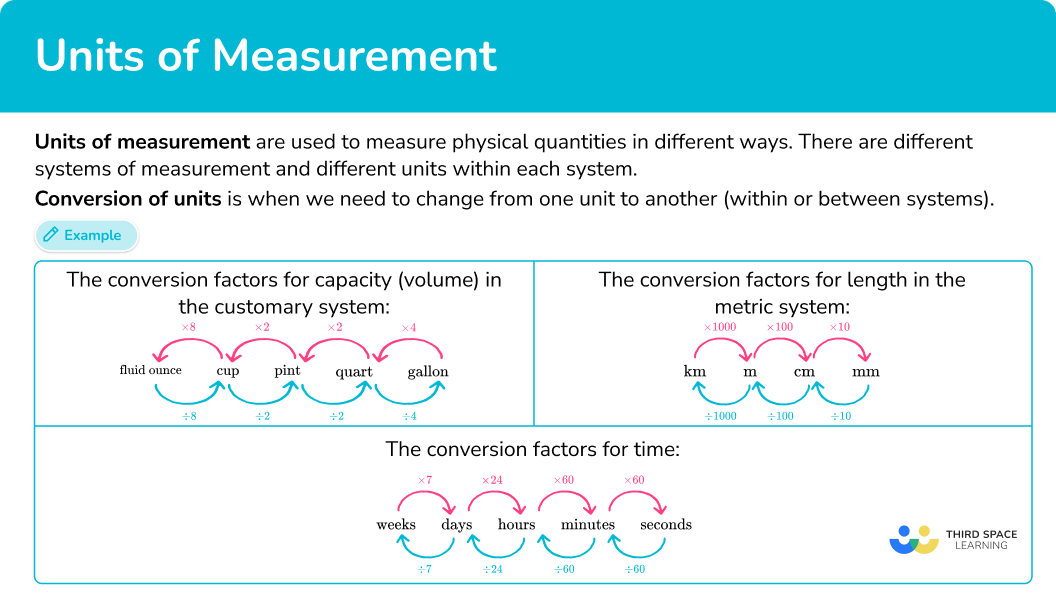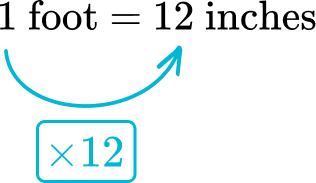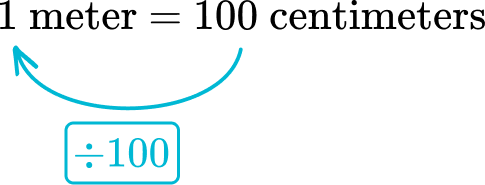Math resources Measurement and data

Units of measurement

# Units of measurement

Here you will learn about units of measurement from the metric system and customary system.

Students will first learn about units of measurement as part of measurement and data in 4 th and 5 th grade and continue to build upon this knowledge in ratios and proportions in 6 th grade.

## What are units of measurement?

Units of measurement are used to measure physical quantities in different ways.

There are different systems of measurement and different units within each system.

Some of the most commonly used units are in the metric system.

Metric units of measurement form part of the international system of units ( SI units) and include units for length, mass and capacity.

For example,

The metric basic unit for length is the meter (m),

The metric basic unit for mass is the gram (g),

The metric basic unit for capacity is the liter (l).

For smaller or larger quantities, you can add a prefix to the base unit. The common ones used are kilo, centi and milli:

Step-by-step guide: Metric units of measurement

The customary system is an older measurement system, but some are still used in everyday life. They include units such as inches, feet, miles (distance) and fluid ounces, cups, pints (volume), ounces and pounds (amount of matter) and Fahrenheit (temperature).

Conversion of units is when we need to change from one unit to another (within or between systems).

For example,

Below are the conversion factors for capacity (volume) in the customary system.

Convert 24 pints into gallons.

There are 8 \text { pints} in 1 \text { gallon.} This is the conversion factor. To convert from pints to gallons you need to divide.

So, 24 \text { pints } \div 8=3 \text { gallons. }

Step-by-step guide: Conversion of measurement units

Converting metric units is when we convert between different metric units of measurement by multiplying or dividing by powers of 10.

For example,

Convert 400 meters into kilometers.

There are 1000 \, m in 1 \, km. This is the conversion factor. To convert from m to km you need to divide.

So, 400 \mathrm{~m} \div 1,000=0.4 \mathrm{~km}.

Step-by-step guide: Converting metric units

Converting units of time is when we need to change from one unit to another within the system of time.

For example,

Some of the standard units of measurement of time and their conversions are shown below.

How many minutes are there in 3 hours?

There are 60 minutes in 1 hour.

So, 3 \text { hours } \times 60=180 \text { minutes. }

Step-by-step guide: Converting units of time

### What are units of measurement?## Common Core State Standards

How does this relate to 4 th grade math?

• Grade 4 – Measurement and Data (4.MD.A.1)
Know relative sizes of measurement units within one system of units including km, m, cm; kg, g; lb, oz.; l, ml; hr, min, sec. Within a single system of measurement, express measurements in a larger unit in terms of a smaller unit. Record measurement equivalents in a two-column table.

For example, know that 1 \, ft is 12 times as long as 1 \, in. Express the length of a 4 \, ft snake as 48 \, in. Generate a conversion table for feet and inches listing the number pairs (1, 12), (2, 24), (3, 36), …

• Grade 4 – Measurement and Data (4.MD.A.2)
Use the four operations to solve word problems involving distances, intervals of time, liquid volumes, masses of objects, and money, including problems involving simple fractions or decimals, and problems that require expressing measurements given in a larger unit in terms of a smaller unit. Represent measurement quantities using diagrams such as number line diagrams that feature a measurement scale.

• Grade 5 – Measurement and Data (5.MD.A.1)
Convert among different-sized standard measurement units within a given measurement system (example, convert 5 \, cm to 0.05 \, m ), and use these conversions in solving multi-step, real world problems.

• Grade 5 – Measurement and Data (6.RP.A.3d)
Use ratio reasoning to convert measurement units; manipulate and transform units appropriately when multiplying or dividing quantities.

## How to use units of measurement

There are a lot of different units of measurement. For more specific step-by-step guides, check out the measurement pages linked in the “What are units of measurement?” section above or read through the examples below.

## Units of measurement examples

### Example 1: units of length

What is the most appropriate metric unit to measure the length of a fly?

1. Decide if the measurement is length, mass, capacity, or time.

Length is measured in metric units based on the meter (m).

2Consider the size.

Thinking about the size of a fly, a meter (about the length of a baseball bat) is too large, and a centimeter (the width of a fingernail) is too large.

3Write down the most reasonable choice.

Millimeters (about the width of the tip of a pencil) is the most appropriate unit to measure the length of a fly.

### Example 2: units of mass

What is the most appropriate metric unit to measure the weight of a grain of salt?

Weight is a unit of mass, so you need to consider the metric measurement units based on the gram (g).

A kilogram (about the weight of a cantaloupe) is too big. A gram (each about the weight of a paper clip) is too big.

A milligram (about the weight of a snowflake) is the most appropriate unit of measurement for the mass of a grain of salt.

### Example 3: converting units of volume (capacity)

Convert 6 gallons to quarts.

Since quarts is the desired unit, you need the conversion factor between gallons and quarts:

1 \text { gallon }=4 \text { quarts }

The conversion factor for gallons to quarts is 4.

Look at the relationship in the original conversion.

From gallons to quarts, the relationship is multiplying by 4.

Multiply 6 gallons by 4 :

6 \text { gallons } \times 4=24 \text { quarts }

6 \text { gallons }=24 \text { quarts }

### Example 4: converting units of time

Convert 2,700 seconds to minutes.

60 \text { seconds }=1 \text { minute }

The conversion factor for minutes and seconds is 60.

Look at the relationship in the original conversions.

From seconds to minutes, the relationship is dividing by 60.

Divide 2,700 seconds by 60 :

2,700 \text { seconds } \div 60=45 \text { minutes }

2,700 \text { seconds }=45 \text { minutes }

### Example 5: converting length

The distance from Lorient, France to Quimper, France is 68.8 \, km. Convert the distance to meters.

1,000 \mathrm{~m}=1 \mathrm{~km}

Look at the relationship in the original conversion.

From meters to kilometers, the relationship is multiplying by 1,000.

Multiply 68.8 kilometers by 1,000 :

68.8 \text { kilometers } \times 1,000=68,800 \text { meters }

68.8 \mathrm{~km}=68,800 \mathrm{~m}

### Example 6: convert days to hours using conversion table

How many hours are there in 5 days?

1 \text { day }=24 \text { hours }

2 \text { days }=24+24=48 \text { hours }

3 \text { days }=48+24=72 \text { hours }

4 \text { days }=72+24=96 \text { hours }

5 \text { days }=96+24=120 \text { hours }

There are 120 hours in 5 days.

### Teaching tips for units of measurement

• When teaching certain conversions, consider which multiples will be necessary for students to use. Such as the multiples of 12 for converting between feet and inches or the multiples of 10 when converting from centimeters to millimeters.

• Start by giving students real world measuring experiences. Include measuring the same object in multiple units, like the volume of water bottle in cups, pints, and quarts, or activities that require students to decide the most appropriate units to use. When students have a solid foundation for how to measure metric and customary units and their relative sizes, they are ready to start converting.

• Students should have access to the conversion factors within the different systems. They can be included in a student’s interactive math notebook for reference or be clearly displayed in the classroom.

• If students struggle converting, give them access to a conversion calculator or unit converter and require them to explain the conversion and the calculations. Once students have explained enough examples, they will begin to solve problems on their own.

### Easy mistakes to make

• Confusing when to multiply or divide
To decide whether to multiply or divide, look at the original conversion. See if the number representing the measurement changes by multiplication or division. This will show you how to convert. The general rule for this is:
• if you are going from larger units to smaller units – multiply
• if you are going from smaller units to larger units – divide

• Confusing the conversion units for US customary units
The customary units are not base 10 conversion units, like the metric system, making them harder to remember.
For example,
Some of the direct capacity conversions include 8, 4, and 2.

• Fractions of an hour
Remember that time (above seconds) is not a decimal system based on 10. A quarter of an hour is 15 minutes. But one quarter as a decimal is 0.25.
For example,
3.25 hours is 3 hours and 15 minutes.
Similarly, 1 \, \cfrac{1}{2} days is 1 day and 12 hours.

• Using the one dimensional factor for the conversion factor of area
Area is a two-dimensional measurement, so it does not convert in the same way as a one-dimensional measurement.
For example,
1 centimeter = 10 millimeters, but 1 square centimeter 10 square millimeters.
1 yard = 3 feet, but 1 square yard 3 square feet.

• Using the one dimensional factor for the conversion factor of volume
Volume is a three-dimensional measurement, so it does not convert in the same way as a one-dimensional measurement.
For example,
1 meter = 100 centimeters, but 1 cubic meter 100 cubic centimeters
1 yard = 3 feet, but 1 cubic yard 3 cubic feet

### Practice units of measurement questions

1. Choose the most reasonable metric unit to measure:

The weight of a beankilogramsmilligramspoundslitersYou need a unit for mass – so liters can be ruled out; it is a measurement of capacity.

You need a metric unit – so pounds can be ruled out; it is a customary unit.

A kilogram is about the weight of 3 chapter books, so it is too large.

A milligram is about the weight of a small feather. Milligrams is the most reasonable unit to measure the weight of a bean.

2. Choose the most reasonable metric unit to measure:

The length of a truckmetersgramsfeetkilometersYou need a unit for length – so grams can be ruled out; it is a measure of mass.

You need a metric unit – so feet can be ruled out; it is a customary unit.

A meter is about the length of a baseball bat. Meters is the most reasonable unit to measure the length of a truck.

3. Convert 36 feet to inches:

3 \text { inches }12 \text { inches }432 \text { inches }1,296 \text { inches }The conversion factor between feet and inches: 1 \text { foot }=12 \text { inches }Multiply 36 feet by 12 :

36 \text { feet } \times 12=432 \text { inches }

36 \text { feet }=432 \text { inches }

4. Convert 255 liters to milliliters:

2.55 \text { milliliters }25.5 \text { milliliters }25,500 \text { milliliters }255,000 \text { milliliters }Multiply 255 liters by 1,000 :

255 \text { liters } \times 1,000=255,000 \text { milliliters }

255 \text { liters }=255,000 \text { milliliters }

5. Convert: 781 \, cm to m.

781 \mathrm{~m}7.81 \mathrm{~m}7,810 \mathrm{~m}0.781 \mathrm{~m}Look at the relationship in the original conversion.From centimeters to meters, the relationship is dividing by 100.

Divide 781 centimeters by 100 :

781 \text { centimeters } \div 100=7.81 \text { meters }

6. How many hours are in 1 week?

168 \text { hours }48 \text { hours }24 \text { hours }225 \text { hours }You can convert from weeks to days to hours.

There are 7 days in a week.

There are 24 hours in 1 day, so multiply days by 24.

7 \text { days } \times 24=168 \text { hours }

There are 168 hours in 1 week.

## Units of measurements FAQs

Yes. For example, the SI System includes the SI units of measurement: ampere – which measures electric current; kelvin – which measures thermodynamic temperature; mole – which measures the amount of substance; and candela – which measures luminous intensity.

What is the speed of light?

The speed of light, another unit of measurement, is 300,000 kilometers per second in a vacuum (empty space).

What is the difference between a ton and a tonne?

The definition varies, depending on the system. A tonne, also known as a metric ton, is 1,000 kilograms. As an imperial unit, part of the imperial system, it is equal to 2,240 pounds. In the customary system, a ton is 2,000 pounds.

## Still stuck?

At Third Space Learning, we specialize in helping teachers and school leaders to provide personalized math support for more of their students through high-quality, online one-on-one math tutoring delivered by subject experts.

Each week, our tutors support thousands of students who are at risk of not meeting their grade-level expectations, and help accelerate their progress and boost their confidence.